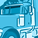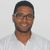# New to Qlik Sense

If you’re new to Qlik Sense, start with this Discussion Board and get up-to-speed quickly.

Announcements
Welcome to Qlik Community! Check out our new navigation! FIND OUT MORE
cancel
Showing results for
Did you mean:Partner - Creator II

## Calculate Time (in mins) between Two Flags

Hello,

Hope all is well with everyone.

We are working on a fleet management system and have a scenario in which we have to sum the time (in mins) for Engine On and Engine Off.

We have 3 fields - TunID, DateTime, di1

What we want to do is for each TunID we would to calculate the time between

* when di1=1 and di1=0 - Engine On
* when di1=0 and di1=1 - Engine Off

Please find attached the excel sheet showing the logic and how it is calculated

 TunID DateTime di1 1001 12/20/19 11:45 PM 1 1001 12/21/19 3:47 AM 1 1001 12/21/19 4:59 PM 1 1001 12/21/19 5:08 PM 0 1001 12/21/19 8:18 PM 0 1001 12/21/19 9:31 PM 1 1001 12/21/19 9:46 PM 1 1001 12/22/19 2:07 AM 1 1001 12/22/19 2:27 AM 1 1001 12/22/19 2:31 AM 1 1001 12/22/19 4:14 AM 1 1001 12/22/19 9:18 AM 0 1001 12/22/19 9:36 AM 0 1001 12/22/19 9:49 AM 1 1001 12/22/19 10:19 AM 1 1001 12/22/19 10:34 AM 1 1001 12/22/19 11:58 AM 1 1001 12/22/19 1:19 PM 0

will appreciate if someone can share the logic how we can do it in Qlik.  For each block of 1&0 we have to look at the first occurrence of 1 and first occurrence of 0.  How can we use the Peek in this regards.

Labels (1)
• ### Qlik Sense calculate time between rows

4 RepliesMVP

Regards,
Taoufiq ZARRA

"Please LIKE posts and "Accept as Solution" if the provided solution is helpful "

(you can mark up to 3 "solutions") 😉MVP

To build a perfect logic please provide data with expected output for multiple TunIDPartner - Creator II
Author

Kush,

The logic will be the same for each of the tunid.  You can just replicate the same and change the tunid.

Thanks.MVP

T1:
TunID,
DateTime,
di1
FROM [lib://Qlik web]
(html, utf8, embedded labels, table is @1);

T2:
if((TunID= Previous(TunID) and di1=0 and Previous(di1)=1) or
(TunID= Previous(TunID) and di1=1 and Previous(di1)=0), (DATE_DIFF-Previous(DATE_DIFF))*24*60,Peek(DATE_DIFF_MIN1)) as DATE_DIFF_MIN1;
if((RowNo()=1 and di1=0) or (TunID<>Previous(TunID) and di1=0),'First Occurance of 0',
if(TunID= Previous(TunID) and di1=0 and Previous(di1)=1, 'First Occurance of 0 after 1',
if((RowNo()=1 and di1=1) or (TunID<>Previous(TunID) and di1=1),'First Occurance of 1',
if(TunID= Previous(TunID) and di1=1 and Previous(di1)=0,'First Occurance of 1 after 0',Peek(Status))))) as Status,

if((TunID= Previous(TunID) and di1=0 and Previous(di1)=1) or (RowNo()=1 and di1=1) or (TunID<>Previous(TunID) and di1=1), 'Moving',
if(TunID= Previous(TunID) and di1=1 and Previous(di1)=0 or (RowNo()=1 and di1=0) or (TunID<>Previous(TunID) and di1=0), 'Stopped',Peek(Flag))) as Flag,

if((TunID= Previous(TunID) and di1=0 and Previous(di1)=1) or (RowNo()=1 and di1=1) or (TunID<>Previous(TunID) and di1=1), DateTime,
if(TunID= Previous(TunID) and di1=1 and Previous(di1)=0 or (RowNo()=1 and di1=0) or (TunID<>Previous(TunID) and di1=0), DateTime,Peek(DATE_DIFF))) as DATE_DIFF
Resident T1
Order by TunID,DateTime;

Drop Table T1;

T3: